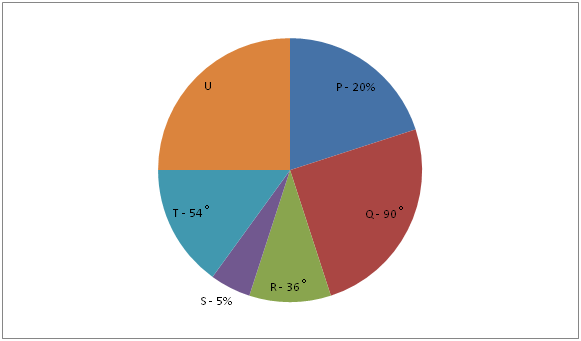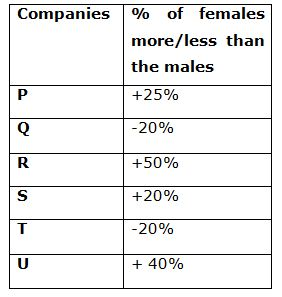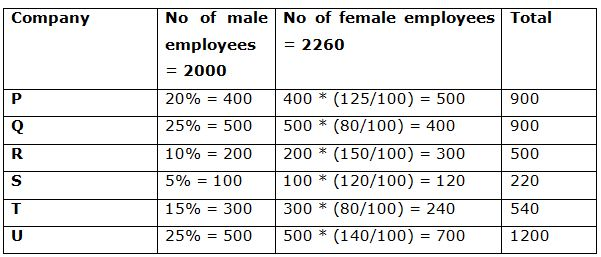# LIC HFL Assistant Manager Quantitative Aptitude Questions – (Day-01)

Dear Aspirants, Our IBPS Guide team is providing new series of Quantitative Aptitude Questions for LIC HFL Assistant Manager 2019 so the aspirants can practice it on a daily basis. These questions are framed by our skilled experts after understanding your needs thoroughly. Aspirants can practice these new series questions daily to familiarize with the exact exam pattern and make your preparation effective.

Check here for LIC HFL Mock Test 2019

Check here for IBPS RRB PO Mains Mock Test 2019

Check here for IBPS RRB Clerk Mains Mock Test 2019

### Click Here to Subscribe Crack High Level Puzzles & Seating Arrangement Questions PDF 2019 Plan

[WpProQuiz 6883]

Directions (1 – 5): Study the following information carefully and answer the given questions.

The pie chart given below shows the distribution of the number of male employees in percentage or in absolute value or in degree in six different companies in 2017.

42.857% of the number of female employees in company U is 300.Note: (+ ve) sing indicates females more than males and (-ve) sign indicates females less than males.

1) Find the total number of employees in company S

a) 240

b) 220

c) 300

d) 180

e) 200

2) If x number of female employees joins in company Q in the year 2018 and the number of female employees in company Q in the year 2018 is 40% of the number of employees in company U in the year 2017. What is the value of x?

a) 60

b) 40

c) 120

d) 80

e) 100

3) What is the ratio of the total male employees in company R and T together to the difference between the female employees in company U and P?

a) 4: 5

b) 3: 7

c) 5: 2

d) 8: 9

e) None of these

4) Number of female employees in company S is what percentage of the average number of male employees in company Q, R and U together?

a) 25%

b) 36%

c) 24%

d) 32%

e) None of these

5) If the ratio of the number of employees like Veg to Non-veg in company T is 2: 1 and the number of female employees in company T like Veg is 33.33% more than the number of female employees in company S. What is the ratio of the number of female to male employees like Non – veg?

a) 5: 4

b) 3: 2

c) 6: 7

d) 4: 7

e) 2: 5

6) A is 8 years older than B and B is 12 years younger than C. If the sum of the ages of A, B and C is 80 years, then what is B’s age after 10 years?

a) 20 years

b) 30 years

c) 28 years

d) 22 years

e) None of these

7) If the certain number of students in the class and the average weight of the class is x kg. If after one month 5 students of the class is reduced their weight by 4 kg, then the average weight of the class is reduced by 2 kg. What is the total number of students in the class?

a) 20

b) 15

c) 10

d) 8

e) None of these

8) The ratio of the income of A and B is 3: 2 and the ratio of their expenditure is 6: 5. If the ratio of the income to savings of A is 3: 1, then what is the ratio of the savings of A to B?

a) 2: 1

b) 2: 3

c) 3: 1

d) 3: 2

e) None of these

9) Ratio of the length to breadth of the rectangular floor is 2: 1. If Rs.1440 is required to paint the floor at the rate of Rs.5 per square meter, then what is the difference between the length and breadth of the rectangular floor?

a) 10 cm

b) 12 cm

c) 14 cm

d) 16 cm

e) None of these

10) Sunil spends 60% of his income on education fee. He spends 30% of remaining on food and 50% of the remaining on shopping. If he left with him Rs.2800, then what is the amount he spends on education fee?

a) Rs.12000

b) Rs.20000

c) Rs.24000

d) Rs.28000

e) None of these

Directions (1 – 5):

Percentage of males in company P = 20%

Percentage of males in company Q = 90˚ * 100/360 = 25%

Percentage of males in company R = 36˚ * 100/360 = 10%

Percentage of males in company S = 5%

Percentage of males in company T = 54˚ * 100/360 = 15%

Percentage of males in company U = 100% – (20% + 25% + 10% + 5% + 15%)

= 100% – 75% = 25%

Number of female employees in company U = 300/42.857 * 100

= 300 * 7/3 = 700

Number of male employees in company U = 700 *(100/140) = 500

Total number of male employees in all the companies together = 500 * (100/25)

= 2000Total number of employees in company S = 100 + 120 = 220

Number of female employees in company Q in the year 2018

= 1200 * 2/5 = 480

Number of female employees joins in company Q in the year 2018 = 480 – 400 = 80

Required ratio = (200 + 300): (700 – 500)

= 500: 200 = 5: 2

Required percentage = 120/[(500 + 200 + 500)/3] * 100

= 120/400 * 100 = 30%

Total employees in company T = 300 + 240 = 540

Number of employees like Veg = 540 * 2/3 = 360

Number of employees like Non – veg = 540 * 1/3 = 180

Number of female employees like veg = 120 * (100 + 33.33)/100

= 120 * (4/3) = 160

Number of female employees like Non – veg = 240 – 160 = 80

Number of male employees like Non – veg = 180 – 80 = 100

Required ratio = 100: 80 = 5: 4

Direction (6-10) :

A – B = 8

C – B = 12

A + B + C = 80

B + 8 + B + 12 + B = 80

3B = 60

B = 20 years

After 10 years B’s age = 20 + 10 = 30 years

Number of students = y

Total weight of the class = xy

Total weight of the class after reduced the weight = xy – 5 * 4 = xy – 20

(x – 2) * y = xy – 20

xy – 2y = xy – 20

2y = 20

y = 10

Income of A = 3x

Income of B = 2x

Expenditure of A = 6y

Expenditure of B = 5y

A’s savings = 3x – 6y

A’s savings = 1/3 *  3x = x

3x – 6y = x

2x = 6y

x = 3y

B’s savings = 2x – 5y = 2x – 5 * (x/3)

= 6x – 5x/3 = x/3

Required ratio = x: x/3 = 3: 1

Area of the rectangle = l * b

2x * x = 1440/5

x = 12 m

Length = 2 * 12 = 24 m

Difference = 24 – 12 = 12 cm

Education fee = 60/100 * x

Remaining = 40/100 * x

Food = 40/100 * x * 30/100 = 12x/100

Remaining = 40x/100 – 12x/100 = 28x/100

Shopping = 28x/100 * 50/100 = 14x/100

Remaining = 14x/100

14x/100 = 2800

x = 20000

Education fee = (20000/100) * 60 = 12000